随着中国汽车市场的持续发展以及消费者环保观念的不断成熟，对汽车轮胎的各项性能要求越来越高，尤其是静音性能。上海市于2018年底颁布并实施了新的交通噪音治理方案——全天禁止噪音超过80分贝的九座以下客车在本市道路（高速公路除外）行驶。为了更好的满足消费者对轮胎的静音性需求，通伊欧轮胎于2019年1月正式推出针对中国市场研发的静音舒适高性能轿车轮胎PROXES Comfort MC1，并同时为SUV车型推出同款静音舒适高性能SUV轮胎PROXES Comfort MC1 SUV。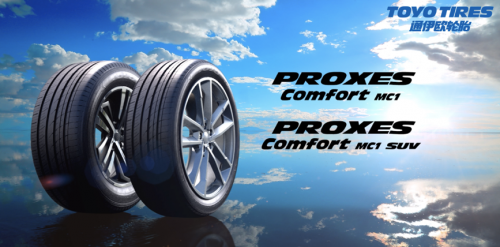通伊欧静音舒适高性能轿车轮胎PROXES Comfort MC1是目前通伊欧运用最多科技的一款轮胎产品，通过复杂花纹排列和独特静音壁设计的强强联合，静音效果得以显著提升，经中国轮胎标签认证，滚动噪声低至68分贝，而低于70分贝均是人耳可接受的舒适范围。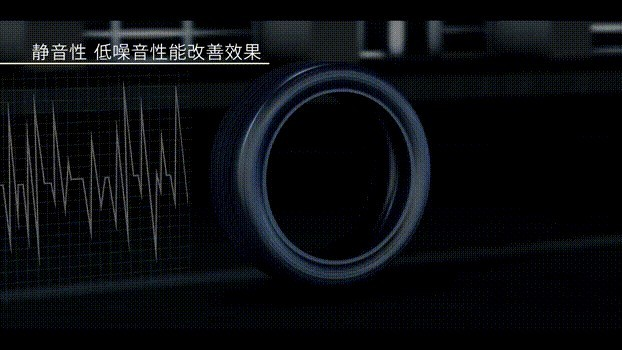PROXES Comfort MC1拥有复杂的胎面花纹设计。内外侧不同比例的花纹块排列设计、内侧横向细纹槽设计、外侧可变节距的花纹块设计都可以有效分散噪音，减少声音叠加，从而降低轮胎与地面摩擦产生的噪音；独特的静音壁设计运用了手枪消音器原理，花纹沟内壁上的密集锯齿状构造能扰乱空气流通有效降低噪音输出。不仅外部设计到位，PROXES Comfort MC1胎体内部也有静音设计。无接头带束层保护层，提高轮胎高速耐久性； 噪音缓冲层，大幅降低路面噪音。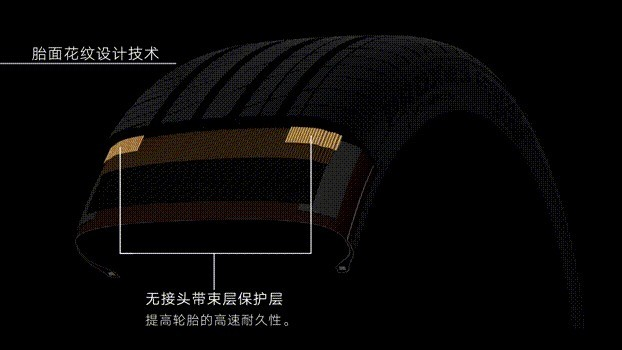在城市道路中行驶，大家往往希望轮胎既拥有低滚动阻力省油，又希望轮胎在雨天行驶中能保证高制动和高操控性能，让通伊欧静音舒适高性能轿车轮胎PROXES Comfort MC1通过纳米平衡技术在一款轮胎上实现了并存。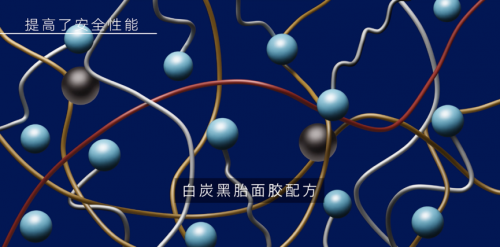PROXES Comfort MC1采用纳米平衡技术，在胎面配方中增加了二氧化硅的使用量，低燃耗效果加抓地力聚合物配方，确保轮胎同时拥有低滚动阻力和高湿地性能两大优点。复杂的胎面花纹设计也提升了轮胎的湿地性能和耐偏磨性。PROXES Comfort MC1的4条宽幅主沟槽花纹设计，能有效确保排水性能从而提升湿地通过性；三连锥形与圆周方向花纹条设计，抑制圆周方向花纹块的变动提高耐偏磨性能，同时也确保花纹条块刚性从而提高干地性能；内外两侧3D波纹切槽设计，能有效防止轮胎胎肩偏磨，在轮胎转向时依然提供安全可靠的抓地力。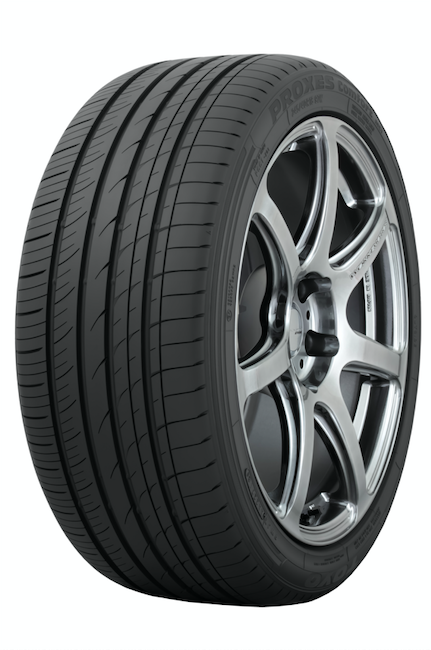此外，PROXES Comfort MC1运用计算机模拟轮胎技术（T-Mode技术），对轮胎花纹沟槽排出路面积水的情况、微小的振动变化、摩擦力、滚动阻力及接地面形状在计算机上进行模拟，辅助设计最佳胎面断面轮廓和最佳的胎面接地压力分布，提升轮胎的安全性能。

轮胎其实并非看似那么简单，它由多种部件组合而成，材质或尺寸的丝毫偏差都会影响轮胎的均一性，造成抖动影响驾乘体验。通伊欧经过研究证实，正圆是在滚动模式下状态最稳定的几何图形，据此通伊欧研发出了高精度新工艺技术——正圆新技术。正圆新技术应用于专为中国车主打造的通伊欧静音舒适高性能轮胎PROXES Comfort MC1和PROXES Comfort MC1 SUV，实现了轮胎的正圆性和均一性，提高了车辆的驾乘舒适感，成功获得中国轮胎标签认证，滚动噪声低至68分贝。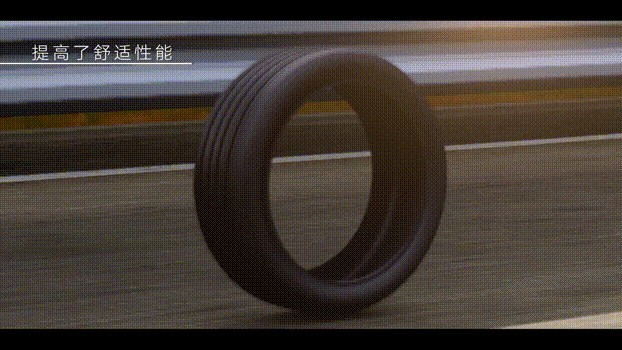在与通伊欧以往轮胎的对比测试结果中，PROXES Comfort MC1在轮胎制动性能和滚动阻力方面都有显著提升， 其湿地制动距离和干地制动距离分别缩短了9%和2%，滚动阻力降低了20%。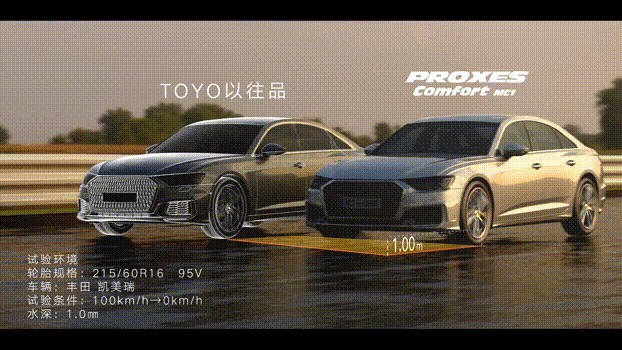通伊欧轮胎以在顶级飘移赛事D1GP的出色表现享誉全球，从赛道到公路，从竞技到生活，凭借新材料和新技术的不断升级，通伊欧从未停止挑战并超越自我。凭借丰富的赛事经验和先进的工艺流程管理，通伊欧不断为中国消费者研制出更高性能的轮胎产品，为广大城市车主带来堪比赛道的极致驾乘体验。

【关于通伊欧轮胎】

通伊欧轮胎（上海）贸易有限公司作为TOYO TIRE株式会社在中国的销售公司，成立于2002年11月。注册资金300万美元。TOYO TIRE投资比例为60%，三菱商事投资比例为40%。公司致力于轿车子午胎和载重子午胎在中国的推广，把日本高技术和优品质的通伊欧轮胎介绍给中国的广大用户。

##### 相关资讯

|分享

•已阅！握爪
•我手滑为你点赞
•128个赞！
•不明觉厉
•阅后既醉
•有钱！任性
•照片太美，我不敢看
•干货！杠杠的
•高大上
•膜拜中！
•请接受我的膝盖
•猫哥/猫妹么么哒
•神吐槽
•我只笑笑不说话
•我想静静
•窒息
##### 资讯相关车系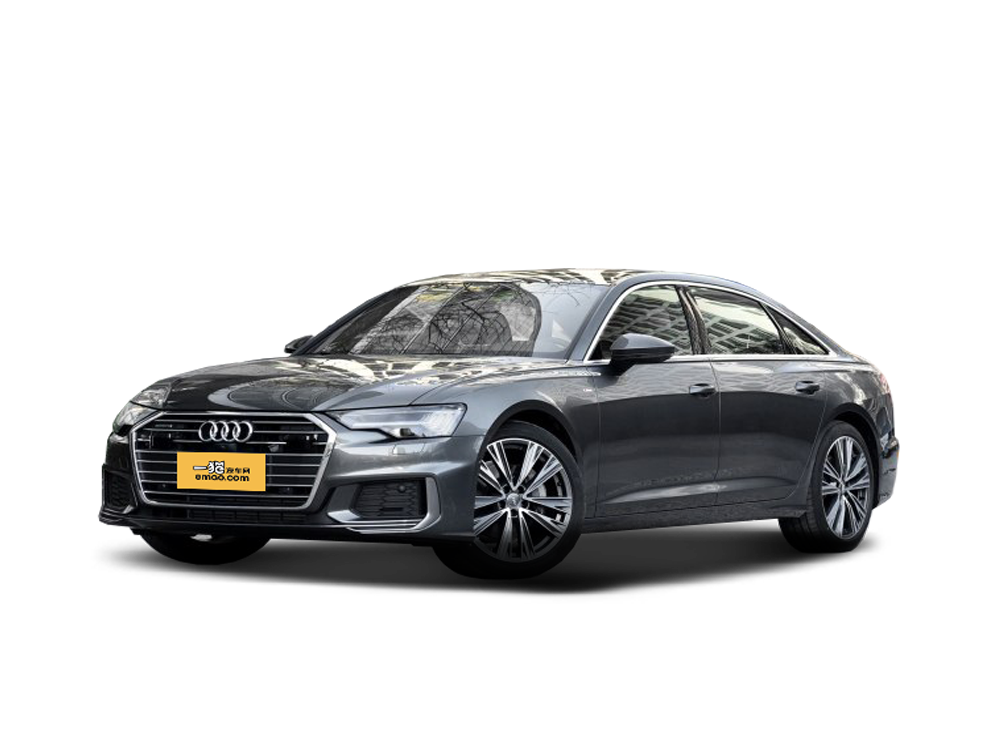##### 热度排行• 阿斯顿·马丁
• 奥迪
• 阿尔法罗密欧
• ALPINA

• 巴博斯
• 宝骏
• 宝马
• 保时捷
• 北汽制造
• 奔驰
• 奔腾
• 本田
• 比亚迪
• 标致
• 别克
• 宾利
• 布加迪
• 北汽威旺
• 北京
• 北汽绅宝
• 北汽幻速
• 北汽新能源
• 宝沃
• 比速汽车
• 北汽道达

• 昌河
• 长安
• 长城
• 长安商用
• 成功汽车
• 长江EV
• 长安轻车型

• 大众
• 道奇
• 东风
• 东风风神
• 东风小康
• 东南
• DS
• 东风风行
• 东风风度
• 东风风光

• 法拉利
• 菲亚特
• 丰田
• 福迪
• 福特
• 福田汽车
• 福汽启腾
• 风诺

• GMC
• 广汽传祺
• 广汽吉奥
• 观致

• 哈飞
• 海格
• 海马
• 华泰
• 黄海
• 恒天
• 红旗
• 哈弗
• 华颂
• 华凯
• 华泰新能源
• 汉腾汽车

• Jeep
• 江淮
• 江铃
• 捷豹
• 金杯
• 九龙
• 吉利汽车
• 金旅
• 金龙
• 江铃集团轻汽
• 江铃集团新能源
• 君马汽车
• 捷途

• 开瑞
• 凯迪拉克
• 科尼赛克
• 克莱斯勒
• KTM
• 卡威
• 凯翼
• 康迪
• 康迪电动汽车集团

• 猎豹汽车
• 兰博基尼
• 劳斯莱斯
• 雷克萨斯
• 雷诺
• 理念
• 力帆
• 莲花汽车
• 林肯
• 铃木
• 陆风
• 路虎
• 路特斯
• 领志
• 领克
• 零跑汽车

• MG
• MINI
• 马自达
• 玛莎拉蒂
• 迈凯伦
• 摩根

• 纳智捷

• 讴歌
• 欧宝
• 欧朗
• 欧拉
• 欧尚汽车

• 帕加尼

• 奇瑞
• 启辰
• 起亚
• 前途
• 庆铃汽车

• 日产
• 荣威
• 瑞麒汽车
• 如虎
• 瑞驰

• 上汽大通
• smart
• 三菱
• 双环
• 双龙
• 斯巴鲁
• 斯柯达
• 萨博
• 思铭
• 赛麟
• SWM斯威汽车

• TESLA
• 泰卡特
• 腾势

• 威麟
• 威兹曼
• 沃尔沃
• 五菱汽车
• 五十铃
• 潍柴英致
• WEY
• 蔚来
• 威马汽车

• 现代
• 雪佛兰
• 雪铁龙
• 西雅特
• 新特汽车
• 小鹏汽车

• 野马汽车
• 一汽
• 依维柯
• 英菲尼迪
• 永源
• 驭胜

• 中华
• 中兴
• 众泰
• 知豆
• 之诺
• 正道汽车
• A
• B
• C
• D
• E
• F
• G
• H
• I
• J
• K
• L
• M
• N
• O
• P
• Q
• R
• S
• T
• U
• V
• W
• X
• Y
• Z

• 阿斯顿·马丁
• 奥迪
• 阿尔法罗密欧
• ALPINA

• 巴博斯
• 宝骏
• 宝马
• 保时捷
• 北汽制造
• 奔驰
• 奔腾
• 本田
• 比亚迪
• 标致
• 别克
• 宾利
• 布加迪
• 北汽威旺
• 北京
• 北汽绅宝
• 北汽幻速
• 北汽新能源
• 宝沃
• 比速汽车
• 北汽道达

• 昌河
• 长安
• 长城
• 长安商用
• 成功汽车
• 长江EV
• 长安轻车型

• 大众
• 道奇
• 东风
• 东风风神
• 东风小康
• 东南
• DS
• 东风风行
• 东风风度
• 东风风光

• 法拉利
• 菲亚特
• 丰田
• 福迪
• 福特
• 福田汽车
• 福汽启腾
• 风诺

• GMC
• 广汽传祺
• 广汽吉奥
• 观致

• 哈飞
• 海格
• 海马
• 华泰
• 黄海
• 恒天
• 红旗
• 哈弗
• 华颂
• 华凯
• 华泰新能源
• 汉腾汽车

• Jeep
• 江淮
• 江铃
• 捷豹
• 金杯
• 九龙
• 吉利汽车
• 金旅
• 金龙
• 江铃集团轻汽
• 江铃集团新能源
• 君马汽车
• 捷途

• 开瑞
• 凯迪拉克
• 科尼赛克
• 克莱斯勒
• KTM
• 卡威
• 凯翼
• 康迪
• 康迪电动汽车集团

• 猎豹汽车
• 兰博基尼
• 劳斯莱斯
• 雷克萨斯
• 雷诺
• 理念
• 力帆
• 莲花汽车
• 林肯
• 铃木
• 陆风
• 路虎
• 路特斯
• 领志
• 领克
• 零跑汽车

• MG
• MINI
• 马自达
• 玛莎拉蒂
• 迈凯伦
• 摩根

• 纳智捷

• 讴歌
• 欧宝
• 欧朗
• 欧拉
• 欧尚汽车

• 帕加尼

• 奇瑞
• 启辰
• 起亚
• 前途
• 庆铃汽车

• 日产
• 荣威
• 瑞麒汽车
• 如虎
• 瑞驰

• 上汽大通
• smart
• 三菱
• 双环
• 双龙
• 斯巴鲁
• 斯柯达
• 萨博
• 思铭
• 赛麟
• SWM斯威汽车

• TESLA
• 泰卡特
• 腾势

• 威麟
• 威兹曼
• 沃尔沃
• 五菱汽车
• 五十铃
• 潍柴英致
• WEY
• 蔚来
• 威马汽车

• 现代
• 雪佛兰
• 雪铁龙
• 西雅特
• 新特汽车
• 小鹏汽车

• 野马汽车
• 一汽
• 依维柯
• 英菲尼迪
• 永源
• 驭胜

• 中华
• 中兴
• 众泰
• 知豆
• 之诺
• 正道汽车
• A
• B
• C
• D
• E
• F
• G
• H
• I
• J
• K
• L
• M
• N
• O
• P
• Q
• R
• S
• T
• U
• V
• W
• X
• Y
• Z

• 阿斯顿·马丁
• 奥迪
• 阿尔法罗密欧
• ALPINA

• 巴博斯
• 宝骏
• 宝马
• 保时捷
• 北汽制造
• 奔驰
• 奔腾
• 本田
• 比亚迪
• 标致
• 别克
• 宾利
• 布加迪
• 北汽威旺
• 北京
• 北汽绅宝
• 北汽幻速
• 北汽新能源
• 宝沃
• 比速汽车
• 北汽道达

• 昌河
• 长安
• 长城
• 长安商用
• 成功汽车
• 长江EV
• 长安轻车型

• 大众
• 道奇
• 东风
• 东风风神
• 东风小康
• 东南
• DS
• 东风风行
• 东风风度
• 东风风光

• 法拉利
• 菲亚特
• 丰田
• 福迪
• 福特
• 福田汽车
• 福汽启腾
• 风诺

• GMC
• 广汽传祺
• 广汽吉奥
• 观致

• 哈飞
• 海格
• 海马
• 华泰
• 黄海
• 恒天
• 红旗
• 哈弗
• 华颂
• 华凯
• 华泰新能源
• 汉腾汽车

• Jeep
• 江淮
• 江铃
• 捷豹
• 金杯
• 九龙
• 吉利汽车
• 金旅
• 金龙
• 江铃集团轻汽
• 江铃集团新能源
• 君马汽车
• 捷途

• 开瑞
• 凯迪拉克
• 科尼赛克
• 克莱斯勒
• KTM
• 卡威
• 凯翼
• 康迪
• 康迪电动汽车集团

• 猎豹汽车
• 兰博基尼
• 劳斯莱斯
• 雷克萨斯
• 雷诺
• 理念
• 力帆
• 莲花汽车
• 林肯
• 铃木
• 陆风
• 路虎
• 路特斯
• 领志
• 领克
• 零跑汽车

• MG
• MINI
• 马自达
• 玛莎拉蒂
• 迈凯伦
• 摩根

• 纳智捷

• 讴歌
• 欧宝
• 欧朗
• 欧拉
• 欧尚汽车

• 帕加尼

• 奇瑞
• 启辰
• 起亚
• 前途
• 庆铃汽车

• 日产
• 荣威
• 瑞麒汽车
• 如虎
• 瑞驰

• 上汽大通
• smart
• 三菱
• 双环
• 双龙
• 斯巴鲁
• 斯柯达
• 萨博
• 思铭
• 赛麟
• SWM斯威汽车

• TESLA
• 泰卡特
• 腾势

• 威麟
• 威兹曼
• 沃尔沃
• 五菱汽车
• 五十铃
• 潍柴英致
• WEY
• 蔚来
• 威马汽车

• 现代
• 雪佛兰
• 雪铁龙
• 西雅特
• 新特汽车
• 小鹏汽车

• 野马汽车
• 一汽
• 依维柯
• 英菲尼迪
• 永源
• 驭胜

• 中华
• 中兴
• 众泰
• 知豆
• 之诺
• 正道汽车
• A
• B
• C
• D
• E
• F
• G
• H
• I
• J
• K
• L
• M
• N
• O
• P
• Q
• R
• S
• T
• U
• V
• W
• X
• Y
• Z